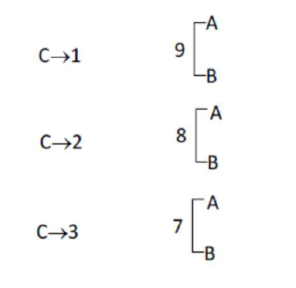# Solve the following

Question:

The students $S_{1}, S_{2}, \ldots, S_{10}$ are to be divided into 3 groups $A, B$ and $C$ such that each group has at least one student and the group $C$ has at most 3 students. Then the total number of possibilities of forming such groups is

Solution:$={ }^{10} C_{1}\left[2^{9}-2\right]+{ }^{10} C_{2}\left[2^{8}-2\right]+{ }^{10} C_{3}\left[2^{7}-2\right]$

$=2^{7}\left[{ }^{10} C_{1} \times 4+{ }^{10} C_{2} \times 2+{ }^{10} C_{3}\right]-20-90-240$

$=128[40+90+120]-350$

$=(128 \times 250)-350$

$=10=31650$